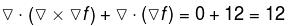Courses

# Vector Calculus NAT Level - 2

## 10 Questions MCQ Test Basic Physics for IIT JAM | Vector Calculus NAT Level - 2

Description
This mock test of Vector Calculus NAT Level - 2 for Physics helps you for every Physics entrance exam. This contains 10 Multiple Choice Questions for Physics Vector Calculus NAT Level - 2 (mcq) to study with solutions a complete question bank. The solved questions answers in this Vector Calculus NAT Level - 2 quiz give you a good mix of easy questions and tough questions. Physics students definitely take this Vector Calculus NAT Level - 2 exercise for a better result in the exam. You can find other Vector Calculus NAT Level - 2 extra questions, long questions & short questions for Physics on EduRev as well by searching above.
*Answer can only contain numeric values
QUESTION: 1

### Evaluate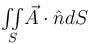where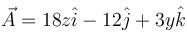and S is the part of the plane 2x + 3y + 6z = 12 which is located in the first octant.

Solution: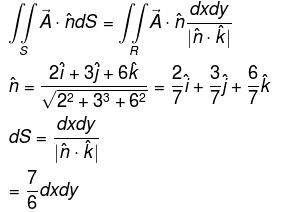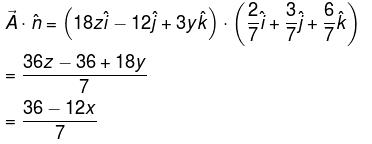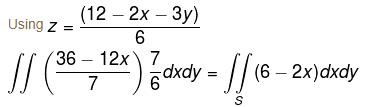= 24

*Answer can only contain numeric values
QUESTION: 2

### Find the value of constant (a + b + c) so that the directional derivative of the function f = axy2 + byz + cz2x3 at the point (1, 2, –1) has maximum magnitude 64 in the direction  parallel to y axis :

Solution: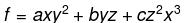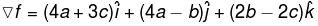lies along y axis
So, 4a + 3c = 0
2b – 2c = 0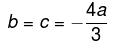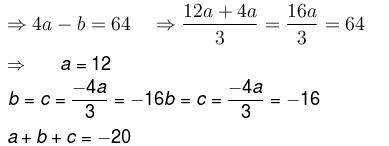*Answer can only contain numeric values
QUESTION: 3

### Evaluate the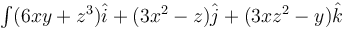along the portion from path (1, 0, 1) to (3, 4, 5) of the curve C, which is the intersection of the surface z2 = x2 + y2 and z = y + 1.

Solution: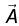can be expressed as gradient of scalar function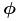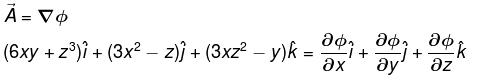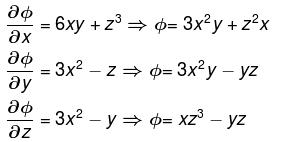written the common term once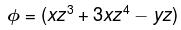The line integral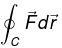along the portion (1, 0, 1) to (3, 4, 5)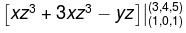= (375 + 108 – 20) – (1)
= 463 – 1
= 462

*Answer can only contain numeric values
QUESTION: 4

The work done by the force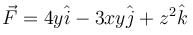in moving a particle over circular path x2 + y2 = 1, z = 0 from (1, 0, 0) to (0, 1, 0) is :

Solution: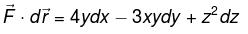on the curve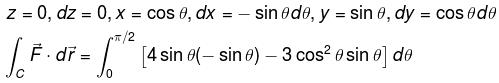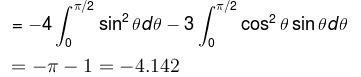*Answer can only contain numeric values
QUESTION: 5

Evaluate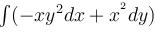where C is the path shown in figure.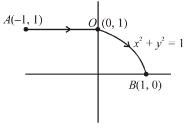Solution:

Path AO
y
= 1
dy = 0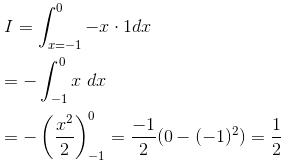Path OB
x
2 + y2 = 1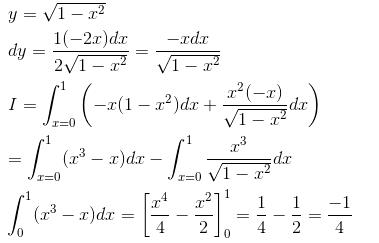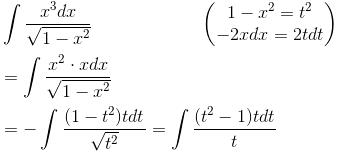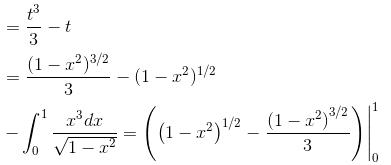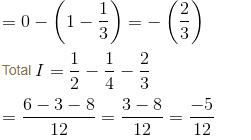= –0.416

*Answer can only contain numeric values
QUESTION: 6

Let C be any curve x2 + y2 + z2 = 4, z > 0 and the vector field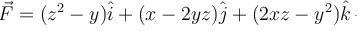(Ans. upto three decimal places)

Solution: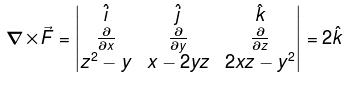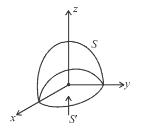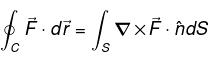Consider a closed surface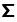consisting of S and S' i.e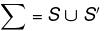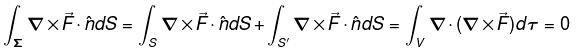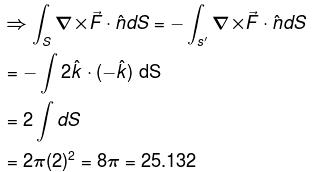*Answer can only contain numeric values
QUESTION: 7

The value of the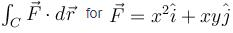and C is the curve y2 = x joining (0, 0) to (1, 1) is (correct upto three decimal places)

Solution: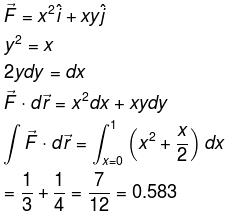*Answer can only contain numeric values
QUESTION: 8

Find the value of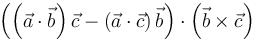Solution:

Let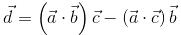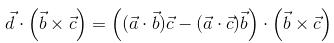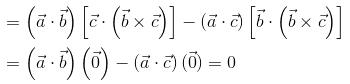*Answer can only contain numeric values
QUESTION: 9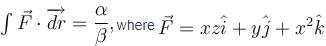along the curve x = sin θ cos θ, y sin2 θ, z = cos θ with θ  increasing from 0 to π/2. Find the value of α + β.

Solution: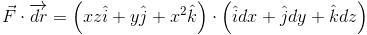xz dx + y dy + xdz
Along the given curve, we have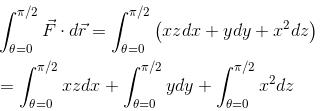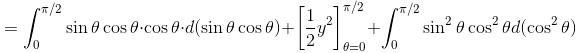Putting values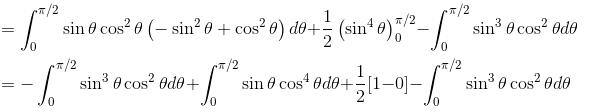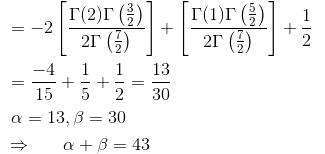*Answer can only contain numeric values
QUESTION: 10

If f(x, y, z) = x2y + y2z + z2x for all (x, y, x) ∈ R3 and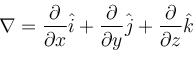then the value of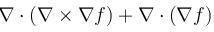at (2, 2, 2) is :

Solution: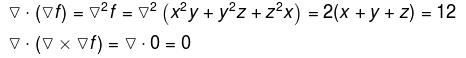Hence,